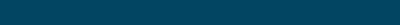### Clinical Biomarker Unit Conversion Tool

Easily convert between Standard International (SI) and Conventional units on your patient's blood lab test results with our free online biomarker unit conversion tool.### Standard International (SI) Unit Converter

Easily convert between the Standard International units used on your patient's blood test results.### Conventional Unit Converter

Easily convert between the Conventional units used on your patient's blood test results.### eAG/A1C Conversion

Estimated Average Glucose (eAG/A1C) calculator.### WBC Absolute Count

Convert between absolute and % values for Total WBCs, Neutrophils, Lymphocytes, Monocytes, Eosinophils, and Basophils.### Free T3 to Reverse T3 Ratio Calculator

Easily calculate the Free T3 to Reverse T3 Ratio using Free T3 and Reverse T3 values.### Free T3 to Free T4 Ratio Calculator

Easily calculate the Free T3 to Free T4 Ratio.### LDL to HDL Ratio Calculator

Easily calculate the LDL to HDL Ratio.### AST to ALT Ratio Calculator

Easily calculate the AST to ALT Ratio.